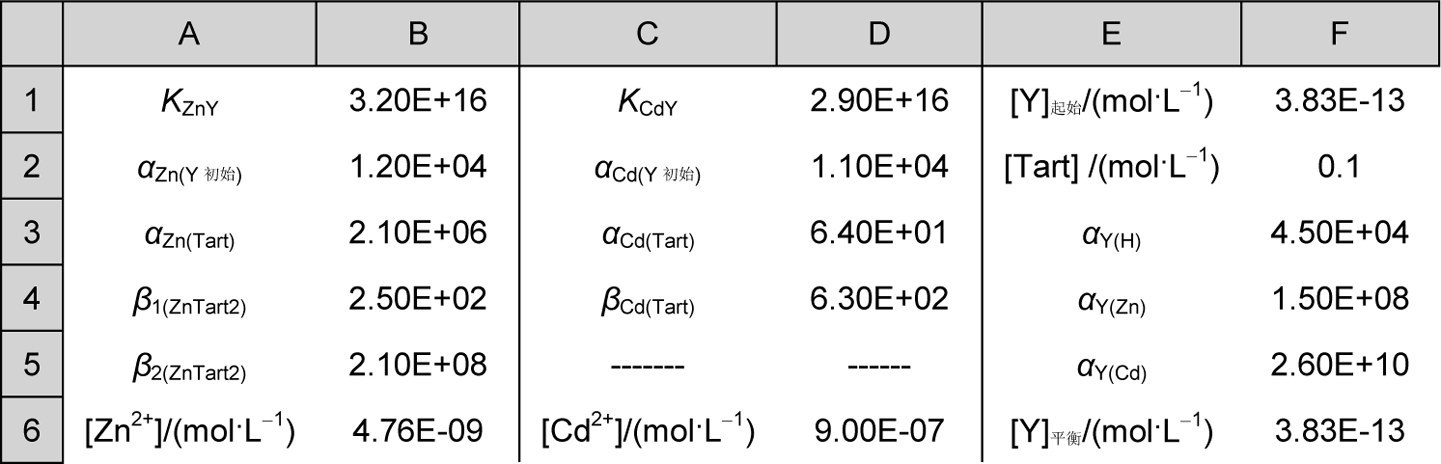1, 1, 2

## Solution for Accurate Calculations in Complicated Coordination Equilibrium System with Excel Iteration Program

1, 1, 2

 基金资助: 陕西师范大学研究生教学改革与研究项目.  GERP-15-09中央高校基本科研业务费专项资金项目.  GK201503003,GK201603104陕西省自然科学基金.  2014JQ2-8048陕西省科技厅社发攻关项目.  2015SF088

 Fund supported: 陕西师范大学研究生教学改革与研究项目.  GERP-15-09中央高校基本科研业务费专项资金项目.  GK201503003,GK201603104陕西省自然科学基金.  2014JQ2-8048陕西省科技厅社发攻关项目.  2015SF088Abstract

The excel iteration program was developed and provided a general approach for accurate calculation of complicated coordination equilibrium systems.

Keywords： Complex coordination equilibrium ; Iteration ; Accurate calculation ; Excel

YUE Xuan-Feng, ZHANG Zhi-Juan, ZHANG Yan-Ni. Solution for Accurate Calculations in Complicated Coordination Equilibrium System with Excel Iteration Program. University Chemistry[J], 2016, 31(8): 89-93 doi:10.3866/PKU.DXHX201510005

### 1.1 涉及单一离子浓度的复杂配位平衡体系的计算

(1)这种做法带来的误差大小很难考查，有时会带来很大的误差，以上例题中简化计算的处理带来的误差并不大，但这只是个特例(简化处理得到的[F-′]sp = 0.2200 mol·L-1，而采用精确处理方法获得的[F-′]sp = 0.2158 mol·L-1)。

(2)在以上做法中做推理时除了必要的逻辑，有时不得不对哪个平衡反应是主要过程进行猜测(比如在金属离子与辅助配位剂生成的各级型体的稳定常数差别不明显的情况下)，这必然增加了判断的不确定性。

(3)有些教材中也提出了逐步逼近法(try-and-error method)，即先通过简化处理获得一个近似的[F-]sp，然后利用这个近似的[F-]sp获得更为准确的αAl(F)，基于此再获得更准确的[F-]sp，采用此法通过多次循环也许可以获得较为准确的结果，但是计算量可能会相当大。

(4)这类处理方法的最大弊端是：并不是所有的复杂多平衡体系都可以这样简化处理，比如：多个平行或者相继平衡反应趋势非常接近的情况。

$\left[ F{{{{-}'}}_{sp}} \right]={{c}_{F}}^{sp}-{{\beta }_{i}}{{\left[ A{{l}^{3+}} \right]}_{sp}}{{\left[ F{-}' \right]}_{sp,初始}}\times i$

${{\left[ A{{l}^{3+}} \right]}_{sp}}={{c}_{A{{l}^{3+}}}}/{{a}_{Al}}_{\left( F \right)}$

${{a}_{Al}}_{\left( F \right)}=1+{{\beta }_{i}}{{\left[ {{F}^{-}} \right]}^{i}}_{sp,初始}$

${{\left[ {{F}^{-}} \right]}_{sp,平衡}}={{\left[ {{F}^{-}}^{\prime } \right]}_{sp,}}/{{a}_{F\left( H \right)}}={{\left[ {{F}^{-}}^{\prime } \right]}_{sp,}}/\left( 1+\left[ H \right]+/{{K}_{a\left( HF \right)}} \right)$

${{\left[ {{F}^{-}} \right]}_{sp,平衡}}=\frac{{{K}_{a\left( HF \right)}}}{\left[ {{H}^{+}} \right]+/{{K}_{a\left( HF \right)}}}\times {{c}_{F}}^{sp}-\frac{{{\beta }_{i}}\times {{c}_{Al}}_{^{3+}}\times {{\left[ {{F}^{-}}^{\prime } \right]}_{sp,初始}}}{1+{{\beta }_{i}}{{\left[ {{F}^{-}}^{\prime } \right]}_{sp,初始}}}$

### 图1(1)进入Excel工作表，在“文件”菜单中选择“选项”命令，然后点击选项“公式”中的“计算选项”，再在“迭代计算”中设置最大迭代次数“1000”(根据误差及有理数解阈值等具体要求确定此数值)，最大允许误差设置为“0”(根据允许误差的要求来设置此数值)。

(2)在单元格B2到F2中依次键入各种已知数值：0.27 (溶液中F-的分析浓度cFsp ，mol·L-1)、0.01 (溶液中Al3+的分析浓度cAl3+ ，mol·L-1)、5.5 (平衡体系的pH)、6.6 × 10-4 (氢氟酸水溶液的解离常数Ka(HF))、0.27 (迭代计算中[F-]sp的初始值[F-]sp,初始，mol·L-1，一般根据单调区间和值阈等给一个合适的初始数值，本例可以取值0.27 mol·L-1)。

(3)第3行到第6行依次填充：各级AlFi的形成常数βi (第3行)；各级AlFi化学式中含有F-的个数(第4行)；αAl(F)的各主要组成式表达式βi[L]i，例如单元格B5中的具体表达式为“=B3*POWER($F$2,B4)”(第5行)；以及各级AlFi形成时消耗的F-的浓度βi[Al3+]sp [F-]isp,初始× i，例单元格B6中的表达式为：“=$C$2/(1+SUM($B$5:$G$5))*B3*POWER($F$2,B4)*B4”(第6行)。

(4)在单元格G2中键入[F-]sp,平衡的表达式，即式(5) (每轮循环计算的终点值)，G2中的具体内容为：“=(B2-SUM(B6:G6))/(POWER(10,-D2)/E2+1)”。按“Enter”键获得来自初始值[F-]sp,初始的第一轮[F-]s,平衡p数值。

(5)再在单元格G2中键入“=F2”，按“Enter”键启动迭代计算。瞬即在单元格F2与G2中同时出现[F-]sp,平衡的数值“0.2158”。

### 1.2 涉及多个离子浓度的复杂配位平衡体系的计算

\left\{ \begin{align} & {{c}_{Zn}}_{^{2+}}=\left[ Z{{n}^{2+}} \right]+\left[ ZnY \right]+\left[ ZnTart \right]+\left[ ZnTar{{t}_{2}} \right]=0.01 \\ & {{c}_{Zd}}_{^{2+}}=\left[ C{{d}^{2+}} \right]+\left[ CdY \right]+\left[ CdTart \right]=0.01 \\ & {{c}_{Y}}=\left[ CdY \right]+\left[ ZnY \right]+\left[ Y \right]+\left[ YH \right]+\left[ Y{{H}_{2}} \right]+\ldots \ldots +\left[ Y{{H}_{6}} \right]=0.01 \\ \end{align} \right.

\left\{ \begin{align} & \left[ Z{{n}^{2+}} \right]\left( {{a}_{Zn\left( Y \right)}}+{{a}_{Zn\left( Tart \right)}}-1 \right)=0.01 \\ & \left[ C{{d}^{2+}} \right]\left( {{a}_{Cd\left( Y \right)}}+{{a}_{Cd\left( Tart \right)}}-1 \right)=0.01 \\ & \left[ Y \right]\left( {{a}_{Y}}_{\left( Zn \right)}+{{a}_{Y}}_{\left( Cd \right)}+{{a}_{Y}}_{\left( H \right)}-2 \right)=0.01 \\ \end{align} \right.

\left\{ \begin{align} & \left[ Z{{n}^{2+}} \right]\left( {{K}_{ZnY}}\left[ Y \right]+{{\beta }_{1}}\left[ Tart \right]+{{\beta }_{1}}{{\left[ Tart \right]}^{2}}+1 \right)=0.01 \\ & \left[ C{{d}^{2+}} \right]\left( {{K}_{CdY}}\left[ Y \right]+\beta \left[ Tart \right]+1 \right)=0.01 \\ & \left[ Y \right]\left( {{K}_{ZnY}}\left[ Z{{n}^{2+}} \right]+{{K}_{CdY}}\left[ C{{d}^{2+}} \right]+{{a}_{Y}}_{\left( H \right)} \right)=0.01 \\ \end{align} \right.

$\left[ Y \right]=0.01{{\left( {{K}_{ZnY}}\left[ Z{{n}^{2+}} \right]+{{K}_{CdY}}\left[ C{{d}^{2+}} \right]+{{a}_{Y}}_{\left( H \right)} \right)}^{-1}}$

{{\left[ Y \right]}_{平衡}}=0.01{{\left( \begin{align} & {{K}_{ZnY}}\frac{0.01}{{{K}_{ZnY}}{{\left[ Y \right]}_{初始}}+{{\beta }_{1}}\left[ Tart \right]+{{\beta }_{1}}{{\left[ Tart \right]}^{2}}+1} \\ & {{K}_{CdY}}\frac{0.01}{{{K}_{CdY}}{{\left[ Y \right]}_{初始}}+\beta \left[ Tart \right]+1+{{a}_{Y}}_{\left( H \right)}} \\ \end{align} \right)}^{-1}}

### 图2(1)设置同2.1节。

(2)在B列、D列和F列分别输入与Zn2+、Cd2+和配位剂有关的常数、浓度及副反应系数等。从B1到B6分别输入KZnYαZn(Y初始) (1+KZnY[Y]初始，具体表达式为“=1+B1*F1”)、αZn(Tart) (β1[Tart]+β2[Tart2]2+1，具体表达式为“=1+B4*F2+B5*POWER(F2,2)”)、β1(ZnTart2)β2(ZnTart2)及[Zn2+] (0.01×(KZnY[Y]+β1[Tart]+β2[Tart]2+1)-1，具体表达式为：“=0.01/(POWER(10,16.5)*F1+POWER(10,6.32)+POWER(10,1.4)+1)”)。

(3)再在单元格F1中键入“=F6”，按“Enter”键启动迭代运算。瞬即在单元格B6、D6及F6中出现[Zn2+]、[Cd2+]和[Y]的数值。

## 参考文献 原文顺序 文献年度倒序 文中引用次数倒序 被引期刊影响因子

/

 〈〉